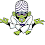# Data Structures and Algorithms Multiple Choice Questions - Set 24

231.       Let the following circular queue can accommodate maximum six elements with the
following data
front = 2             rear = 4
queue = .........; L, M, N, ...., ....
What will happen after ADD O operation takes place?
(A) front = 2       rear = 5
queue = ..........; L, M, N, O, .........
(B) front = 3       rear = 5
queue = L, M, N, O, .........
(C) front = 3      rear = 4
queue = .........; L, M, N, O, .........
(D) front = 2      rear = 4
queue = L, M, N, O, .........
232.       A binary tree of depth “d” is an almost complete binary tree if:
(A) Each leaf in the tree is either at level “d” or at level “d–1”
(B) For any node “n” in the tree with a right descendent at level “d” all the left descendents of “n” that are leaves, are also at level “d”
(C) Both (A) & (B)
(D) None of the above
233.       A linear collection of data elements where the linear node is given by means of pointer is called ...............
(B) Node list
(C) Primitive list
(D) None of these
234.       Representation of data structure in memory is known as:
(A) recursive
(B) abstract data type
(C) storage structure
(D) file structure
235.       If the address of A and A are 1000 and 1010 respectively and each element
occupies 2 bytes then the array has been stored in ............... order.
(A) row major
(B) column major
(C) matrix major
(D) none of these
236.       What data structure can be used to check if syntax has balanced parenthesis?
(A) Queue
(B) Tree
(C) List
(D) Stack
237.       How many binary trees with 3 nodes which when traversed in post order gives the sequence A, B, C is ?
(A) 3
(B) 4
(C) 5
(D) 6
238.       An adjacency matrix representation of a graph cannot contain information of :
(A) nodes
(B) edges
(C) direction of edges
(D) parallel edges
239.       Which data structure represents a waiting line and limits insertions to be made at the back of the data structure and limits removals to be made from the front?
(A) Stack
(B) Queue
(C) Binary tree
240.    Quick sort is also known as .............
(A) Merge sort
(B) Heap sort
(C) Bubble sort
(D) None of these
1.